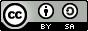# # 线性回归

### # 说明：

1.硬件环境要求： 本文可支持在CPU、GPU下运行 2. Docker镜像支持的CUDA/cuDNN版本： 如果使用了Docker运行Book，请注意：这里所提供的默认镜像的GPU环境为 CUDA 8/cuDNN 5，对于NVIDIA Tesla V100等要求CUDA 9的 GPU，使用该镜像可能会运行失败。 3. 文档和脚本中代码的一致性问题： 请注意：为使本文更加易读易用，我们拆分、调整了train.py的代码并放入本文。本文中代码与train.py的运行结果一致，可直接运行train.py进行验证。

## # 背景介绍## # 效果展示## # 模型概览

### # 模型定义$\hat{Y}$ 表示模型的预测结果，用来和真实值$Y$区分。模型要学习的参数即：$\omega_1, \ldots, \omega_{13}, b$。### # 训练过程

1. 初始化参数，其中包括权重$\omega_i$和偏置$b$，对其进行初始化（如0均值，1方差）。
2. 网络正向传播计算网络输出和损失函数。
3. 根据损失函数进行反向误差传播 （backpropagation），将网络误差从输出层依次向前传递, 并更新网络中的参数。
4. 重复2~3步骤，直至网络训练误差达到规定的程度或训练轮次达到设定值。

## # 数据集

### # 数据集介绍

CRIM 该镇的人均犯罪率 连续值
ZN 占地面积超过25,000平方呎的住宅用地比例 连续值
INDUS 非零售商业用地比例 连续值
CHAS 是否邻近 Charles River 离散值，1=邻近；0=不邻近
NOX 一氧化氮浓度 连续值
RM 每栋房屋的平均客房数 连续值
AGE 1940年之前建成的自用单位比例 连续值
DIS 到波士顿5个就业中心的加权距离 连续值
TAX 全值财产税率 连续值
PTRATIO 学生与教师的比例 连续值
B 1000(BK - 0.63)^2，其中BK为黑人占比 连续值
LSTAT 低收入人群占比 连续值
MEDV 同类房屋价格的中位数 连续值

### # 数据预处理

#### # 属性的归一化

• 过大或过小的数值范围会导致计算时的浮点上溢或下溢。

• 不同的数值范围会导致不同属性对模型的重要性不同（至少在训练的初始阶段如此），而这个隐含的假设常常是不合理的。这会对优化的过程造成困难，使训练时间大大的加长。

• 很多的机器学习技巧/模型（例如L1，L2正则项，向量空间模型-Vector Space Model）都基于这样的假设：所有的属性取值都差不多是以0为均值且取值范围相近的。## # 训练

fit_a_line/train.py演示了训练的整体过程。

### # 配置数据提供器(Datafeeder)

from __future__ import print_function
import numpy
import math
import sys


2. 数据预处理的过程。

BATCH_SIZE = 20

batch_size=BATCH_SIZE)

batch_size=BATCH_SIZE)


feature_names = [
'CRIM', 'ZN', 'INDUS', 'CHAS', 'NOX', 'RM', 'AGE', 'DIS', 'RAD', 'TAX',
'PTRATIO', 'B', 'LSTAT', 'convert'
]
feature_num = len(feature_names)
data = numpy.fromfile(filename, sep=' ') # 从文件中读取原始数据
data = data.reshape(data.shape // feature_num, feature_num)
maximums, minimums, avgs = data.max(axis=0), data.min(axis=0), data.sum(axis=0)/data.shape

for i in six.moves.range(feature_num-1):
data[:, i] = (data[:, i] - avgs[i]) / (maximums[i] - minimums[i]) # six.moves可以兼容python2和python3

ratio = 0.8 # 训练集和验证集的划分比例
offset = int(data.shape*ratio)
train_data = data[:offset]
test_data = data[offset:]

for d in train_data:
yield d[:-1], d[-1:]

batch_size=BATCH_SIZE)

batch_size=BATCH_SIZE)


### # 配置训练程序

x = fluid.layers.data(name='x', shape=, dtype='float32') # 定义输入的形状和数据类型
y = fluid.layers.data(name='y', shape=, dtype='float32') # 定义输出的形状和数据类型
y_predict = fluid.layers.fc(input=x, size=1, act=None) # 连接输入和输出的全连接层

main_program = fluid.default_main_program() # 获取默认/全局主函数
startup_program = fluid.default_startup_program() # 获取默认/全局启动程序

cost = fluid.layers.square_error_cost(input=y_predict, label=y) # 利用标签数据和输出的预测数据估计方差
avg_loss = fluid.layers.mean(cost) # 对方差求均值，得到平均损失


### # Optimizer Function 配置

#克隆main_program得到test_program
#有些operator在训练和测试之间的操作是不同的，例如batch_norm，使用参数for_test来区分该程序是用来训练还是用来测试
#该api不会删除任何操作符,请在backward和optimization之前使用
test_program = main_program.clone(for_test=True)

sgd_optimizer = fluid.optimizer.SGD(learning_rate=0.001)
sgd_optimizer.minimize(avg_loss)



### # 定义运算场所

use_cuda = False
place = fluid.CUDAPlace(0) if use_cuda else fluid.CPUPlace() # 指明executor的执行场所

###executor可以接受传入的program，并根据feed map(输入映射表)和fetch list(结果获取表)向program中添加数据输入算子和结果获取算子。使用close()关闭该executor，调用run(...)执行program。
exe = fluid.Executor(place)



### # 创建训练过程

num_epochs = 100

def train_test(executor, program, reader, feeder, fetch_list):
accumulated = 1 * 
count = 0
outs = executor.run(program=program,
feed=feeder.feed(data_test),
fetch_list=fetch_list)
accumulated = [x_c + x_c for x_c in zip(accumulated, outs)] # 累加测试过程中的损失值
count += 1 # 累加测试集中的样本数量
return [x_d / count for x_d in accumulated] # 计算平均损失



### # 训练主循环

%matplotlib inline
params_dirname = "fit_a_line.inference.model"
feeder = fluid.DataFeeder(place=place, feed_list=[x, y])
exe.run(startup_program)
train_prompt = "train cost"
test_prompt = "test cost"
plot_prompt = Ploter(train_prompt, test_prompt)
step = 0

exe_test = fluid.Executor(place)


paddlepaddle提供了reader机制来读取训练数据。reader会一次提供多列数据，因此我们需要一个python的列表来定义读取顺序。我们构建一个循环来进行训练，直到训练结果足够好或者循环次数足够多。 如果训练迭代次数满足参数保存的迭代次数，可以把训练参数保存到params_dirname。 设置训练主循环

for pass_id in range(num_epochs):
avg_loss_value, = exe.run(main_program,
feed=feeder.feed(data_train),
fetch_list=[avg_loss])
if step % 10 == 0: # 每10个批次记录并输出一下训练损失
plot_prompt.append(train_prompt, step, avg_loss_value)
plot_prompt.plot()
print("%s, Step %d, Cost %f" %
(train_prompt, step, avg_loss_value))
if step % 100 == 0:  # 每100批次记录并输出一下测试损失
test_metics = train_test(executor=exe_test,
program=test_program,
fetch_list=[avg_loss.name],
feeder=feeder)
plot_prompt.append(test_prompt, step, test_metics)
plot_prompt.plot()
print("%s, Step %d, Cost %f" %
(test_prompt, step, test_metics))
if test_metics < 10.0: # 如果准确率达到要求，则停止训练
break

step += 1

if math.isnan(float(avg_loss_value)):
sys.exit("got NaN loss, training failed.")

#保存训练参数到之前给定的路径中
if params_dirname is not None:
fluid.io.save_inference_model(params_dirname, ['x'], [y_predict], exe)


## # 预测

### # 准备预测环境

infer_exe = fluid.Executor(place)
inference_scope = fluid.core.Scope()


### # 预测

def save_result(points1, points2):
import matplotlib
matplotlib.use('Agg')
import matplotlib.pyplot as plt
x1 = [idx for idx in range(len(points1))]
y1 = points1
y2 = points2
l1 = plt.plot(x1, y1, 'r--', label='predictions')
l2 = plt.plot(x1, y2, 'g--', label='GT')
plt.plot(x1, y1, 'ro-', x1, y2, 'g+-')
plt.title('predictions VS GT')
plt.legend()
plt.savefig('./image/prediction_gt.png')


with fluid.scope_guard(inference_scope):
[inference_program, feed_target_names,
fetch_targets] = fluid.io.load_inference_model(params_dirname, infer_exe) # 载入预训练模型
batch_size = 10

infer_feat = numpy.array(
[data for data in infer_data]).astype("float32") # 提取测试集中的数据
infer_label = numpy.array(
[data for data in infer_data]).astype("float32") # 提取测试集中的标签

assert feed_target_names == 'x'
results = infer_exe.run(inference_program,
feed={feed_target_names: numpy.array(infer_feat)},
fetch_list=fetch_targets) # 进行预测
#打印预测结果和标签并可视化结果
print("infer results: (House Price)")
for idx, val in enumerate(results):
print("%d: %.2f" % (idx, val)) # 打印预测结果

print("\nground truth:")
for idx, val in enumerate(infer_label):
print("%d: %.2f" % (idx, val)) # 打印标签值

save_result(results, infer_label) # 保存图片


## # 参考文献

1. https://en.wikipedia.org/wiki/Linear_regression
2. Friedman J, Hastie T, Tibshirani R. The elements of statistical learning[M]. Springer, Berlin: Springer series in statistics, 2001.
3. Murphy K P. Machine learning: a probabilistic perspective[M]. MIT press, 2012.
4. Bishop C M. Pattern recognition[J]. Machine Learning, 2006, 128.